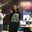Trusted answers to developer questions
Trusted Answers to Developer Questions

Related Tags

nfa

# How to convert Epsilon NFA to NFAAyesha Kanwal

Grokking Modern System Design Interview for Engineers & Managers

Ace your System Design Interview and take your career to the next level. Learn to handle the design of applications like Netflix, Quora, Facebook, Uber, and many more in a 45-min interview. Learn the RESHADED framework for architecting web-scale applications by determining requirements, constraints, and assumptions before diving into a step-by-step design process.### Overview

A nondeterministic finite automaton has zero, one, or more than one transition from one state to another or itself. In epsilon nondeterministic finite automaton, null or epsilon transitions take place from one state to another state.

### The need for an epsilon NFA

An epsilon NFA is formed by a regular expression for a language. This epsilon NFA is then converted to a simple NFA. The obtained NFA is then used for making a deterministic finite automaton.

Note: To learn how to convert an NFA to a DFA, click here.

### Null closure method

Suppose an NFA $$ and $S \subseteq Q$ is a defined set of states. where

• $Q$ is the finite set of states.
• $Σ$ is the input symbols.
• $q_0$ is the start state.
• $δ$ is the transition function.
• $F$ is the final state.

The null closure of $S$ will be the set $Λ(S)$ and can be defined recursively as follows.

1. $S \subseteq Λ(S)$
2. For every $q \in Λ(S)$, $δ(q, Λ) \subseteq Λ(S)$

To convert an epsilon NFA to NFA, the null closure method makes use of the following general set of rules.

1. Find the null closures for each state.
2. For each state, check for the transitions by the null closures obtained, the given input, and then the null closures again. This eliminates any potential null closures that can occur.
3. Make an NFA by the transitions obtained in the previous step. The final state will be all those states that have $F$ in them.

### Example

Consider the following epsilon NFA, $q_0$ is the start state.

Epsilon NFA

#### Step 1: Find null closure of all states

Find the null closure of all the states by the property defined before.

Null Closure
 state set q0 {q0, q1, q3} q1 {q1, q3} q2 {q2} q3 {q3}

#### Step 2: Follow the process of null closure, input, null closure

Implement the process for all states for the given inputs in order.

Transitions
 state 0 1 q0 {q0, q1, q2, q3} - q1 {q2, q3} - q2 - {q1, q3} q3 {q3} -

#### Step 3: Result

Make the NFA through the transitions obtained in the previous step.

NFA

All states that have $q_3$ in their null closure are final states.

RELATED TAGS

nfa

CONTRIBUTORAyesha Kanwal